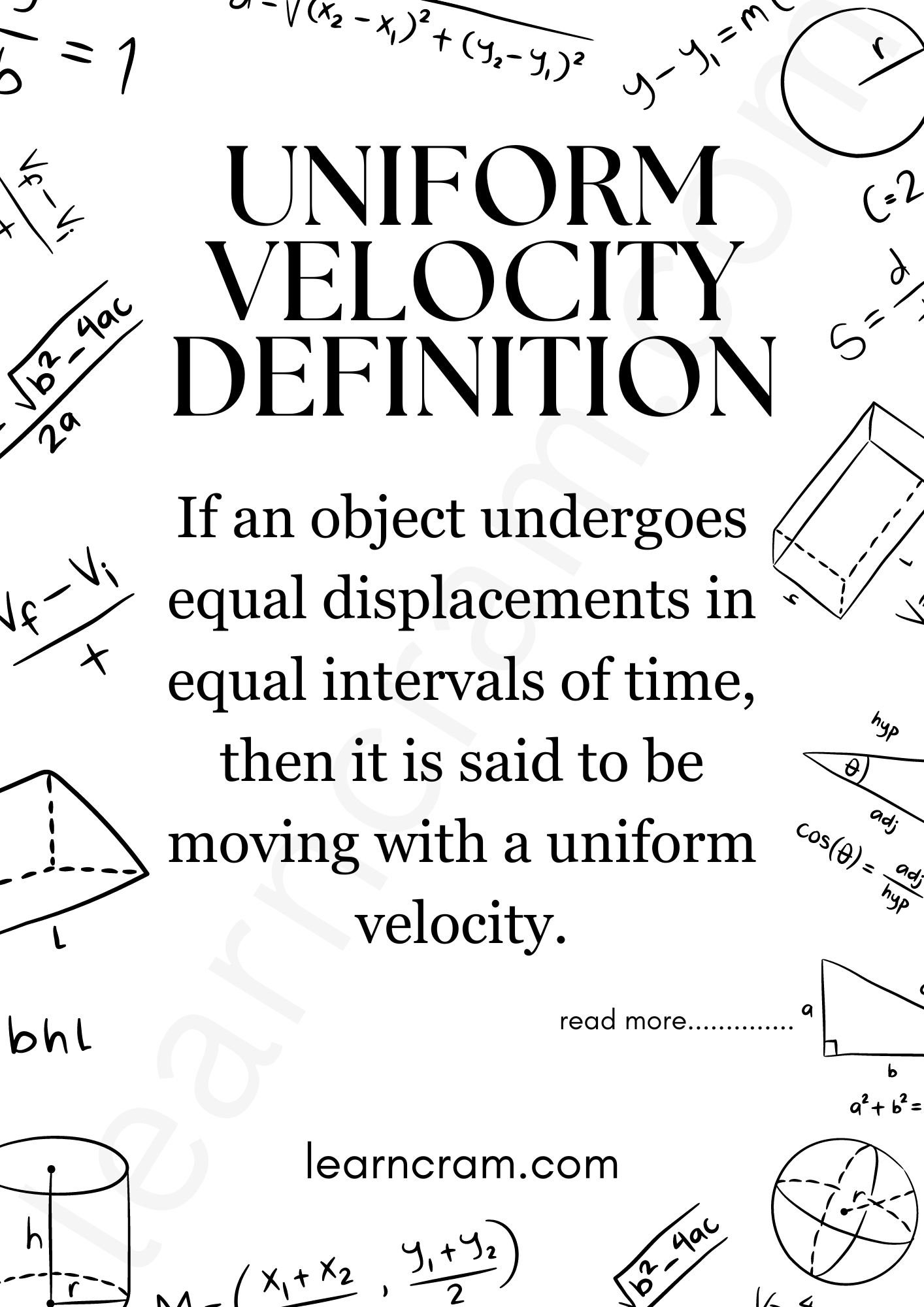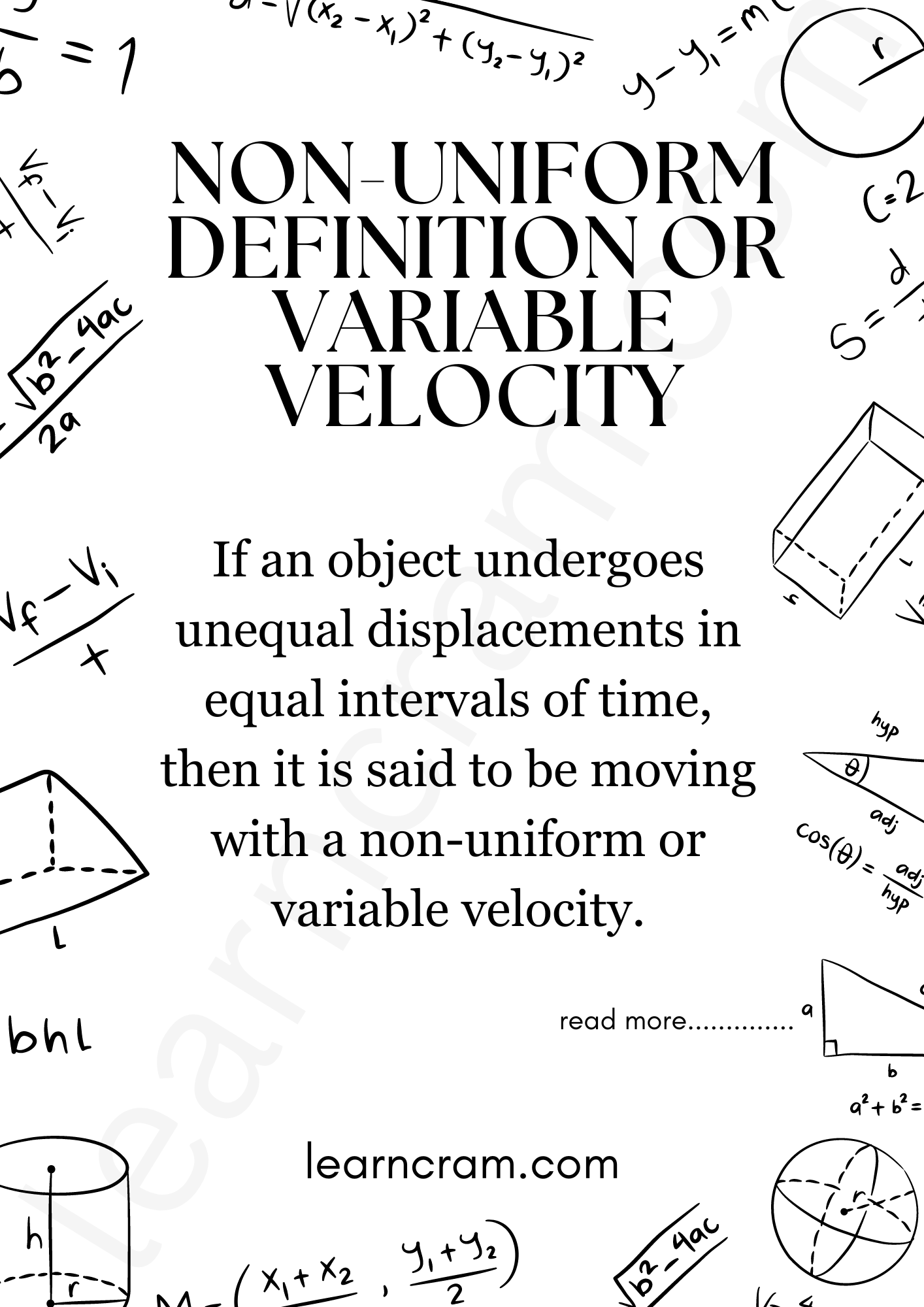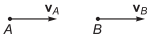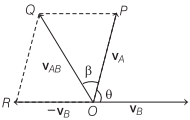# Velocity in Physics | Definition, Types, Formulas, Units – Motion in a Straight Line

Velocity Definition Physics
The time rate of change of displacement of an object in a particular direction is called its velocity.
Velocity Formula in Physics

$$Velocity =\frac{\text { Displacement }}{\text { Time taken }}$$

• Its SI unit is m/s.
• Its dimensional formula is [M°LT-1].
• It is a vector quantity, as it has both, the magnitude and direction.
• The velocity of an object can be positive, zero or negative.

## Velocity | Definition, Type, Formulas, Units – Motion in a Straight Line

We are giving a detailed and clear sheet on all Physics Notes that are very useful to understand the Basic Physics Concepts.

Types of Velocity in Physics

Uniform Velocity Definition:
If an object undergoes equal displacements in equal intervals of time, then it is said to be moving with a uniform velocity.Non-uniform Definition or Variable Velocity:
If an object undergoes unequal displacements in equal intervals of time, then it is said to be moving with a non-uniform or variable velocity.Average Velocity Definition:
The ratio of the total displacement to the total time taken is called average velocity.
Average Velocity Formula Physics

$$Average velocity =\frac{\text { Total displacement }}{\text { Total time taken }}$$

Instantaneous Velocity Definition:
The velocity of a particle at any instant of time is known as instantaneous velocity.
Instantaneous Velocity Formula

$$\text { Instantaneous velocity }=\lim _{\Delta t \rightarrow 0} \frac{\Delta x}{\Delta t}=\frac{d x}{d t}$$

Relative Velocity Definition:
Relative velocity of one object with respect to another object is the time rate of change of relative position of one object with respect to another object.

Relative velocity of object A with respect to object B
VAB =VA – VB
If it is in one dimensional motion, we can treat vectors as scalars just by assigning the positive sign to one direction and negative to others.

when two objects are moving in the same direction, then
VAB = VA – VB
or
VAB = VA – VBWhen two objects are moving in opposite direction, then
VAB = VA + VB
or
VAB = VA + VBWhen two objects are moving at an angle θ, then$$v_{A B}=\sqrt{v_{A}^{2}+v_{B}^{2}-2 v_{A} v_{B} \cos \theta}$$
and
$$\tan \beta=\frac{v_{B} \sin \theta}{v_{A}-v_{B} \cos \theta}$$

Motion in a Straight Line Topics: